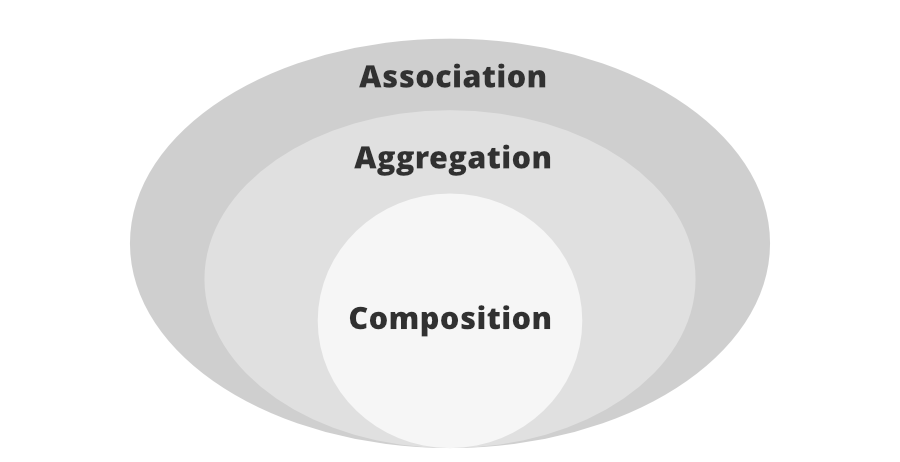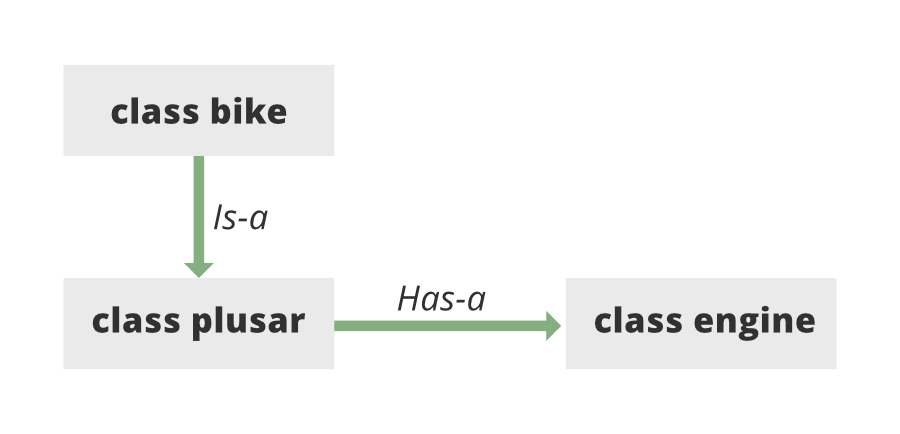# What is Has-A-Relation in Java?

• Difficulty Level : Easy
• Last Updated : 19 Jan, 2021

Association is the relation between two separate classes which establishes through their Objects. Composition and Aggregation are the two forms of association. In Java, a Has-A relationship is otherwise called composition. It is additionally utilized for code reusability in Java. In Java, a Has-A relationship essentially implies that an example of one class has a reference to an occasion of another class or another occurrence of a similar class. For instance, a vehicle has a motor, a canine has a tail, etc. In Java, there is no such watchword that executes a Has-A relationship. Yet, we generally utilize new catchphrases to actualize a Has-A relationship in Java.Has-a is a special form of Association where:

• It represents the Has-A relationship.
• It is a unidirectional association i.e. a one-way relationship. For example, here above as shown pulsar motorcycle has an engine but vice-versa is not possible and thus unidirectional in nature.
• In Aggregation, both the entries can survive individually which means ending one entity will not affect the other entity.

Illustration:This shows that class Pulsar Has-a an engine. By having a different class for the engine, we don’t need to put the whole code that has a place with speed inside the Van class, which makes it conceivable to reuse the Speed class in numerous applications.

In an Object-Oriented element, the clients don’t have to make a big deal about which article is accomplishing the genuine work. To accomplish this, the Van class conceals the execution subtleties from the clients of the Van class. Thus, essentially what happens is the clients would ask the Van class to do a specific activity and the Van class will either accomplish the work without help from anyone else or request that another class play out the activity.

Implementation: Here is the implementation of the same which is as follows:

1. Car class has a couple of instance variable and few methods
2. Maserati is a type of car that extends the Car class that shows Maserati is a Car. Maserati also uses an Engine’s method, stop, using composition. So it shows that a Maserati has an Engine.
3. The Engine class has the two methods start() and stop() that are used by the Maserati class.

Example:

## Java

 `// Java Program to Illustrate has-a relation ` ` `  `// Class1 ` `// Parent class ` `public` `class` `Car { ` ` `  `    ``// Instance members of class Car ` `    ``private` `String color; ` `    ``private` `int` `maxSpeed; ` ` `  `    ``// Main driver method ` `    ``public` `static` `void` `main(String[] args) ` `    ``{ ` `        ``// Creating an object of Car class ` `        ``Car nano = ``new` `Car(); ` ` `  `        ``// Assigning car object color ` `        ``nano.setColor(``"RED"``); ` ` `  `        ``// Assigning car object speed ` `        ``nano.setMaxSpeed(``329``); ` ` `  `        ``// Calling carInfo() over object of Car class ` `        ``nano.carInfo(); ` ` `  `        ``// Creating an object of Maserati class ` `        ``Maserati quattroporte = ``new` `Maserati(); ` ` `  `        ``// Calling MaseratiStartDemo() over ` `        ``// object of Maserati class ` `        ``quattroporte.MaseratiStartDemo(); ` `    ``} ` ` `  `    ``// Methods implementation ` ` `  `    ``// Method 1 ` `    ``// To set the maximum speed of car ` `    ``public` `void` `setMaxSpeed(``int` `maxSpeed) ` `    ``{ ` `        ``// This keyword refers to current object itself ` `        ``this``.maxSpeed = maxSpeed; ` `    ``} ` ` `  `    ``// Method 2 ` `    ``// To set the color of car ` `    ``public` `void` `setColor(String color) ` `    ``{ ` `        ``// This keyword refers to current object ` `        ``this``.color = color; ` `    ``} ` ` `  `    ``// Method 3 ` `    ``// To display car information ` `    ``public` `void` `carInfo() ` `    ``{ ` `        ``// Print the car information - color and speed ` `        ``System.out.println(``"Car Color= "` `+ color ` `                           ``+ ``" Max Speed= "` `+ maxSpeed); ` `    ``} ` `} ` ` `  `// Class2 ` `// Child class ` `// Helper class ` `class` `Maserati ``extends` `Car { ` ` `  `    ``// Method in which it is shown ` `    ``// what happened with the engine of Puslar ` `    ``public` `void` `MaseratiStartDemo() ` `    ``{ ` `        ``// Creating an object of Engine type ` `        ``// using stop() method ` `        ``// Here, MaseratiEngine is name of an object ` `        ``Engine MaseratiEngine = ``new` `Engine(); ` `        ``MaseratiEngine.start(); ` `        ``MaseratiEngine.stop(); ` `    ``} ` `} ` ` `  `// Class 3 ` `// Helper class ` `class` `Engine { ` ` `  `    ``// Method 1 ` `    ``// To start a engine ` `    ``public` `void` `start() ` `    ``{ ` `        ``// Print statement when engine starts ` `        ``System.out.println(``"Started:"``); ` `    ``} ` ` `  `    ``// Method 2 ` `    ``// To stop a engine ` `    ``public` `void` `stop() ` `    ``{ ` `        ``// Print statement when engine stops ` `        ``System.out.println(``"Stopped:"``); ` `    ``} ` `}`

Output

```Car Color= RED Max Speed= 150
Started:
Stopped:```

My Personal Notes arrow_drop_up
Recommended Articles
Page :